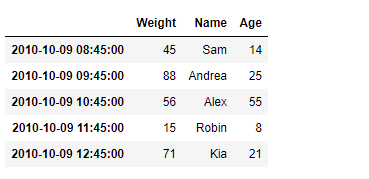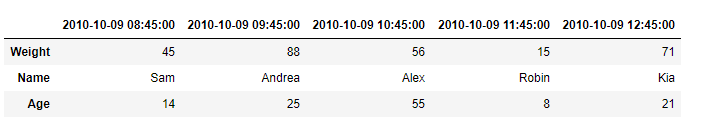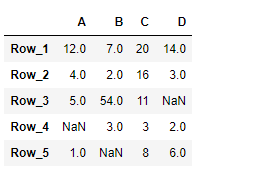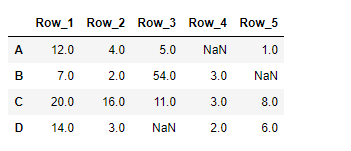# Python | Pandas DataFrame.transpose

• Last Updated : 21 Feb, 2019

Pandas DataFrame is a two-dimensional size-mutable, potentially heterogeneous tabular data structure with labeled axes (rows and columns). Arithmetic operations align on both row and column labels. It can be thought of as a dict-like container for Series objects. This is the primary data structure of the Pandas.

Pandas` DataFrame.transpose()` function transpose index and columns of the dataframe. It reflect the DataFrame over its main diagonal by writing rows as columns and vice-versa.

Syntax: DataFrame.transpose(*args, **kwargs)

Parameter :
copy : If True, the underlying data is copied. Otherwise (default), no copy is made if possible.
*args, **kwargs : Additional keywords have no effect but might be accepted for compatibility with numpy.

Returns : The transposed DataFrame

Example #1: Use `DataFrame.transpose()` function to find the transpose of the given dataframe.

 `# importing pandas as pd ` `import` `pandas as pd ` ` `  `# Creating the DataFrame ` `df ``=` `pd.DataFrame({``'Weight'``:[``45``, ``88``, ``56``, ``15``, ``71``], ` `                   ``'Name'``:[``'Sam'``, ``'Andrea'``, ``'Alex'``, ``'Robin'``, ``'Kia'``], ` `                   ``'Age'``:[``14``, ``25``, ``55``, ``8``, ``21``]}) ` ` `  `# Create the index ` `index_ ``=` `pd.date_range(``'2010-10-09 08:45'``, periods ``=` `5``, freq ``=``'H'``) ` ` `  `# Set the index ` `df.index ``=` `index_ ` ` `  `# Print the DataFrame ` `print``(df) `

Output :Now we will use `DataFrame.transpose()` function to find the transpose of the given dataframe.

 `# return the transpose ` `result ``=` `df.transpose() ` ` `  `# Print the result ` `print``(result) `

Output :As we can see in the output, the `DataFrame.transpose()` function has successfully returned the transpose of the given dataframe.

Example #2: Use `DataFrame.transpose()` function to find the transpose of the given dataframe.

 `# importing pandas as pd ` `import` `pandas as pd ` ` `  `# Creating the DataFrame ` `df ``=` `pd.DataFrame({``"A"``:[``12``, ``4``, ``5``, ``None``, ``1``],  ` `                   ``"B"``:[``7``, ``2``, ``54``, ``3``, ``None``],  ` `                   ``"C"``:[``20``, ``16``, ``11``, ``3``, ``8``],  ` `                   ``"D"``:[``14``, ``3``, ``None``, ``2``, ``6``]})  ` ` `  `# Create the index ` `index_ ``=` `[``'Row_1'``, ``'Row_2'``, ``'Row_3'``, ``'Row_4'``, ``'Row_5'``] ` ` `  `# Set the index ` `df.index ``=` `index_ ` ` `  `# Print the DataFrame ` `print``(df) `

Output :Now we will use `DataFrame.transpose()` function to find the transpose of the given dataframe.

 `# return the transpose ` `result ``=` `df.transpose() ` ` `  `# Print the result ` `print``(result) `

Output :As we can see in the output, the `DataFrame.transpose()` function has successfully returned the transpose of the given dataframe.

My Personal Notes arrow_drop_up
Recommended Articles
Page :